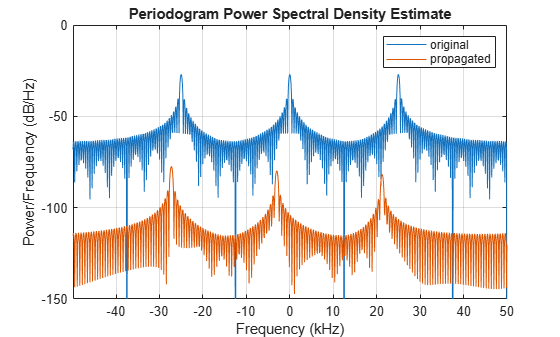## Free-Space Propagation of Wideband Signals

Propagate a wideband signal with three tones in an underwater acoustic with constant speed of propagation. You can model this environment as free space. The center frequency is 100 kHz and the frequencies of the three tones are 75 kHz, 100 kHz, and 125 kHz, respectively. Plot the spectrum of the original signal and the propagated signal to observe the Doppler effect. The sampling frequency is 100 kHz.

Note: This example runs only in R2016b or later. If you are using an earlier release, replace each call to the function with the equivalent `step` syntax. For example, replace `myObject(x)` with `step(myObject,x)`.

```c = 1500; fc = 100e3; fs = 100e3; relfreqs = [-25000,0,25000];```

Set up a stationary radar and moving target and compute the expected Doppler.

```rpos = [0;0;0]; rvel = [0;0;0]; tpos = [30/fs*c; 0;0]; tvel = [45;0;0]; dop = -tvel(1)./(c./(relfreqs + fc));```

Create a signal and propagate the signal to the moving target.

```t = (0:199)/fs; x = sum(exp(1i*2*pi*t.'*relfreqs),2); channel = phased.WidebandFreeSpace(... 'PropagationSpeed',c,... 'OperatingFrequency',fc,... 'SampleRate',fs); y = channel(x,rpos,tpos,rvel,tvel);```

Plot the spectra of the original signal and the Doppler-shifted signal.

```periodogram([x y],rectwin(size(x,1)),1024,fs,'centered') ylim([-150 0]) legend('original','propagated');```For this wideband signal, you can see that the magnitude of the Doppler shift increases with frequency. In contrast, for narrowband signals, the Doppler shift is assumed constant over the band.